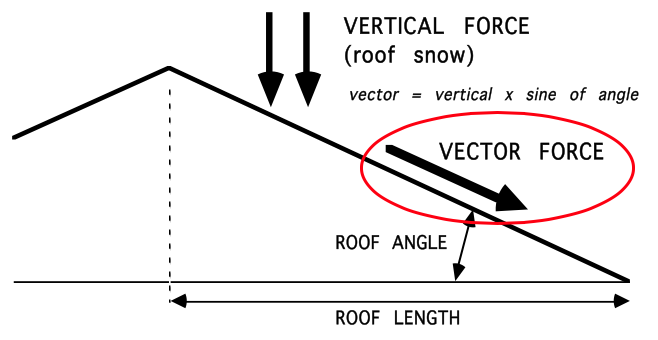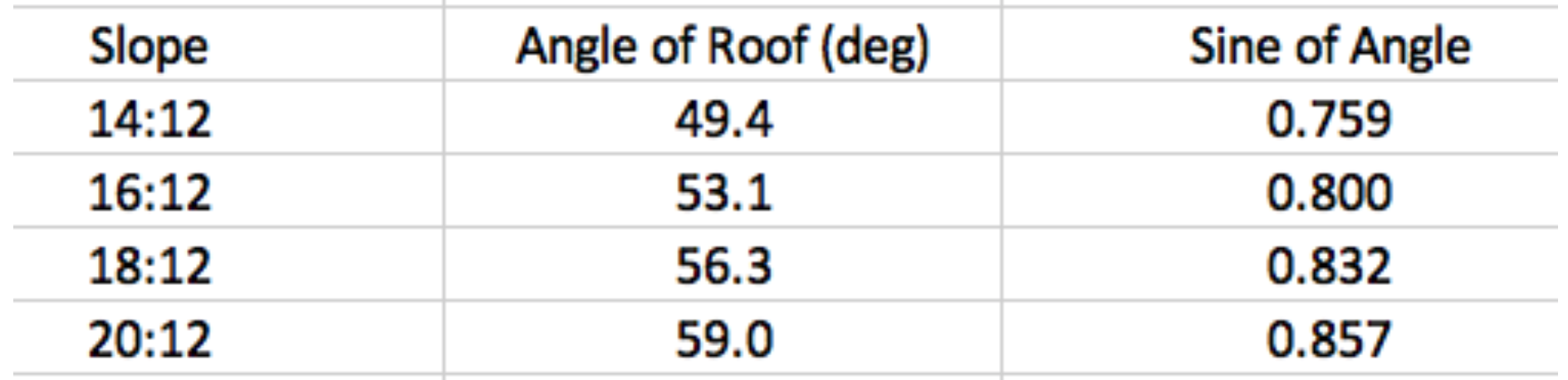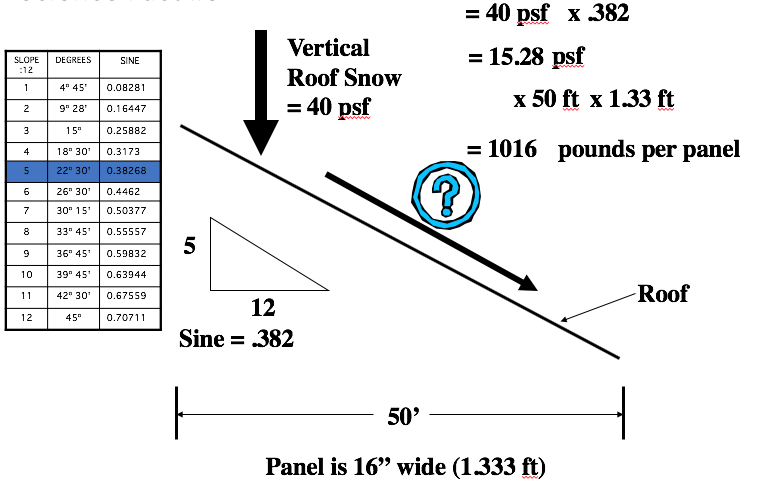# How to calculate snow retention on roofs over 12/12 pitch.

## Our online snow calculator is a very helpful tool when designing a snow retention system but only goes up to a 12:12 (45º) pitch. In the event the roof is steeper than 12:12, some hand-math will be involved to properly engineer a system.

The force of snow on any rooftop can be mathematically calculated—and should be for any snow guard system. It is the same math that runs our online calculator.

Here is how it works:To determine the angular (or “vector”) force manually:

1. Multiply the vertical weight, or roof snow load (in psf) by the sine of the roof angle. There are some tools that follow to determine the sine of any roof angle.

Roof Snow (psf) x Sine (of roof angle) = Vector Force (psf)

2. Take the resulting figure and multiply by the roof length (in feet) and panel width (in feet). The resulting figure is the total force (in pounds per panel) that the system must withstand.

Vector Force x Roof Length (ft) x Panel Width (ft) = Vector Force (pounds per panel)

3. Now, use our allowable clamp load data to determine the number of rows of snow retention needed.

Please see the tools and illustrations below to help walk you through the process:

Use the table below to determine the sine of various roof slopes above 12:12:An illustration of this calculation on a 5:12 roof is as follows:Be aware that any snow retention system is less effective at such steep slopes as being discussed in this FAQ. “Slab avalanche” probabilities increase with steeper slopes, especially in heavy snow areas. This is a phenomenon where a deep bank of snow shears somewhere in its midsection. Such possibility increases with steeper slopes and greater depths of snowpack. Site plans should consider implementing “drop zones” in such cases.